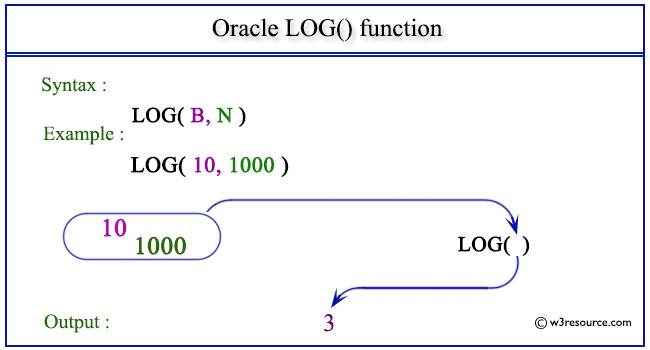# Oracle LOG() function

## Description

The Oracle LOG() function is used to return the logarithm, base B of N. The base can be any positive value other than 0 or 1 and the number can be any positive value.
The function takes any numeric or nonnumeric data type (can be implicitly converted to a numeric data type) as an argument.
If any argument is BINARY_FLOAT or BINARY_DOUBLE, then the function returns BINARY_DOUBLE. Otherwise, the function returns NUMBER.

Syntax:

` LOG(B, N);`

Argument:

Name Description
B Indicates the base of N.
N A number.

Pictorial Presentation of LOG() functionExample: LOG() function using base of a number system

The statement below returns the natural logarithm of 1000 divided by the natural logarithm of 10.

``````SELECT LOG(10,1000) FROM dual;
```
```

Here is the result.

```LOG(10,1000)
------------
3
```

Previous: LN
Next: MOD

﻿